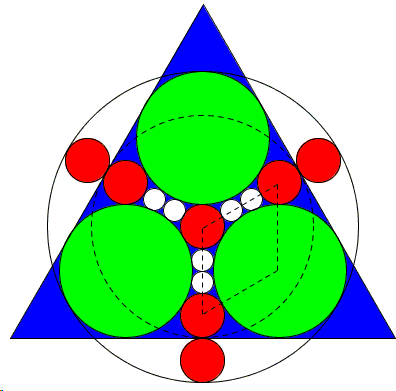# A Sangaku by a Teen

It is said that during the Edo period hanging sangaku in temples and shrines was so popular that even women and children partook of the activity. While I am skeptical that sangaku was so popular as to involve a significant slice of the population, some sangaku have indeed been created by children. Here's one example.In a blue equilateral triangle, three green circles of radius a, four red circles of radius b, and six white circles of radius c touch each other as shown. If R is the radius of the outer circle, and r is the radius of the dashed circle, find c in terms of r.

This sangaku has been hung by Tanabe Shigetoshi, aged fifteen, in the year 1865 at the Meiseirinji temple in Ogaki City, Gifu prefecture.

The sangaku has a very simple solution, although it was anything but simple to come up with such a configuration.

### References

1. H. Fukagawa, A. Rothman, Sacred Mathematics: Japanese Temple Geometry, Princeton University Press, 2008, p. 97In a blue equilateral triangle, three green circles of radius a, four red circles of radius b, and six white circles of radius c touch each other as shown. If R is the radius of the outer circle, and r is the radius of the dashed circle, find c in terms of r.

### Solution

By direct inspection

 r = 3b + 4c, R = 5b + 4c, R = b + 2a, a + b = 2b + 4c.

Solving these simultaneously we see that b = 2c, a = 6c, and r = 10c.## Sangaku